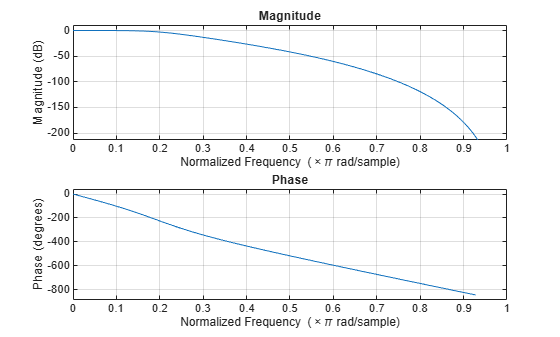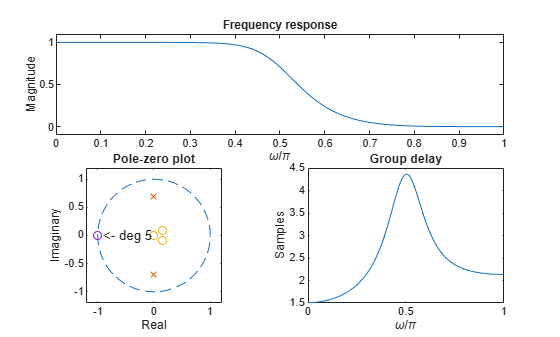# maxflat

Generalized digital Butterworth filter design

## Syntax

[b,a] = maxflat(n,m,Wn)
b = maxflat(n,'sym',Wn)
[b,a,b1,b2] = maxflat(n,m,Wn)
[b,a,b1,b2,sos,g] = maxflat(n,m,Wn)
[___] = maxflat(n,m,Wn,designflag)

## Description

example

[b,a] = maxflat(n,m,Wn) returns the coefficients b and a of a lowpass Butterworth filter with normalized cutoff frequency Wn.
b = maxflat(n,'sym',Wn) returns the coefficients b of a symmetric FIR Butterworth filter. n must be even.
[b,a,b1,b2] = maxflat(n,m,Wn) returns two polynomials b1 and b2 whose product is equal to the numerator polynomial b (that is, b = conv(b1,b2)).
[b,a,b1,b2,sos,g] = maxflat(n,m,Wn) returns the second-order sections representation of the filter as the filter matrix sos and the gain g.

example

[___] = maxflat(n,m,Wn,designflag) specifies the option to display the filter design as a table, plot, or both using designflag. You can use any of the output combinations from previous syntaxes.

## Examples

collapse all

Design a generalized Butterworth filter with normalized cutoff frequency $0.2\pi$ rad/s. Specify a numerator order of 10 and a denominator order of 2. Visualize the frequency response of the filter.

n = 10; m = 2; Wn = 0.2; [b,a] = maxflat(n,m,Wn); fvtool(b,a)Design a generalized Butterworth filter with normalized cutoff frequency 0.5$\pi$ rad/s. Specify a numerator order of 8 and a denominator order of 2. Display the design table and the plots of the filter characteristics.

n = 8; m = 2; Wn = 0.5; b = maxflat(n,m,Wn,'both');
 Table: L M N wo_min/pi wo_max/pi 8.0000 0 2.0000 0 0.2707 7.0000 1.0000 2.0000 0.2707 0.3710 6.0000 2.0000 2.0000 0.3710 0.4581 5.0000 3.0000 2.0000 0.4581 0.5419 4.0000 4.0000 2.0000 0.5419 0.6290 3.0000 5.0000 2.0000 0.6290 0.7293 2.0000 6.0000 2.0000 0.7293 1.0000## Input Arguments

collapse all

Numerator coefficient order, specified as a real positive scalar.

Data Types: single | double

Denominator coefficient order, specified as a real positive scalar.

Data Types: single | double

Normalized cutoff frequency at which the magnitude response of the filter is equal to $1/\sqrt{2}$, specified as a scalar in the range [0, 1], where 1 corresponds to the Nyquist frequency.

Data Types: single | double

Filter design display, specified as one of these values:

• 'trace' for a textual display of the design table used in the design

• 'plots' for plots of the filter magnitude, group delay, and zeros and poles

• 'both' for both the textual display and plots

## Output Arguments

collapse all

Numerator coefficients, returned as a vector.

Denominator coefficients, returned as a vector.

Polynomials, returned as vectors. The product of b1 and b2 is equal to the numerator polynomial b. b1 contains all of the zeros at z = -1, and b2 contains all of the other zeros.

Second-order section coefficients, returned as a matrix.

Gain of the filter, returned as a real-valued scalar.

 Selesnick, Ivan W., and C. Sidney Burrus. “Generalized Digital Butterworth Filter Design.” IEEE® Transactions on Signal Processing 46, no. 6, (June 1998): 1688–94.